8 December 2019

# Free form parametric curves

### How to generate a parametric curve in x(t), y(t), w(t)

Nazca-0.5.8 provides a new way to define your own custom parametric curve. It is based on the existing viper method and it wraps viper functionality such that it handles drawing of all layers in xsections. It automatically adjusts polygon angles at the start and finish of the waveguide shape.

This parametric curve is fundamentally defined as a set of three functions in ‘t’ as x(t), y(t), w(t), for the x and y coordinate of the spine and the width w, where t is a normalized parameter which varies from [0, 1].

The code below provides an example. The function “tapered_bend” is created by the template function Tp_viper(). This stores the funtions x, y, w inside “tapered_bend”. It is possible to add any number of free parameters to the functions x, y and/or w. In this example we have “radius” and “angle” as free parameters. These parameters can  differ per function. All free parameters, and their default values, must be provided when generation “tapered_bend” with Tp_viper(). The free parameters can be used in calls to “tapered_bend”. The use of **kwargs in the function definitions is necessary to make the functions handle the free parameters nicely. Note that in the example below we use xsection ‘Deep’ from demofab.

```# example created by Bright Photonics

import numpy as np
import nazca as nd
import nazca.demofab as demo

# create functions x, y, w for the viper-based mask_element:
"""X as function of t and free parameters."""
return radius * np.cos(t * angle * np.pi / 180)
"""Y as function of t and free parameters."""
return radius * np.sin(t * angle * np.pi / 180)
def w(t, width1=None, width2=None, **kwargs):
"""Width as function of t, width1 and width2 and free parameters."""
return width1 + (width2 - width1) * t

# create the new parametric function using the template Tp_viper():
params = {'radius': 100, 'angle':90} # include defaults for *all* free parameters used in the functions x, y and w.
tapered_bend = nd.Tp_viper(x, y, w, xs='Deep', **params)

# put waveguides:
tapered_bend().put(0)
demo.deep.strt(length=100).put()
tapered_bend(angle=90, width2=20, N=1000).put()
nd.export_gds()```

It is possible to set the number of polygon points per edge via parameter “N”. The calculation of the angle in the begin and end point is based on an infinitesimal step in t of “epsilon” and it can be adjusted too:

```tapered_bend = nd.Tp_viper(
x, y, w, xs='Deep',
N=1000, epsilon=1e-6,
**params)```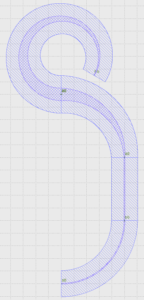## Related Tutorials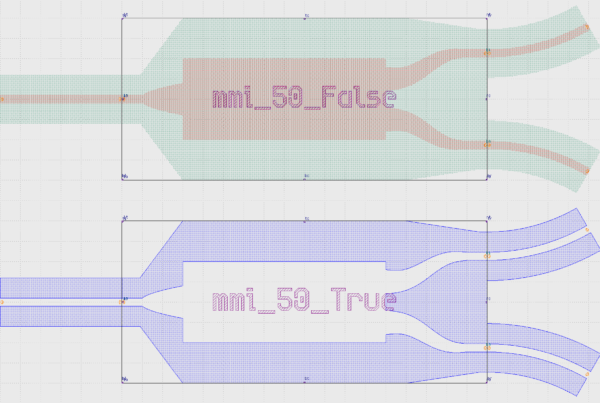In this example we show how to create an inverted MMI with custom interconnects.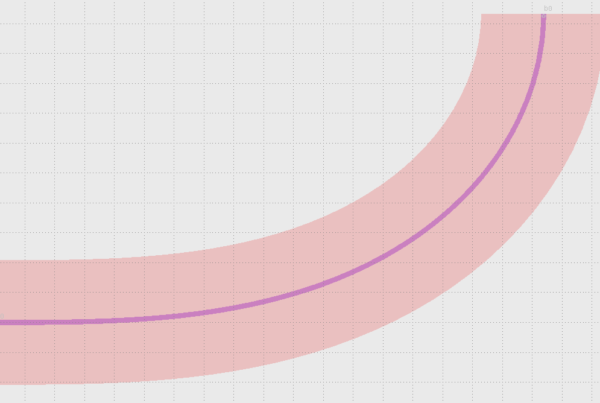In this example we show how to use Euler bends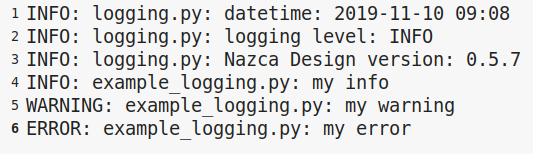In this example we show how to log your layout.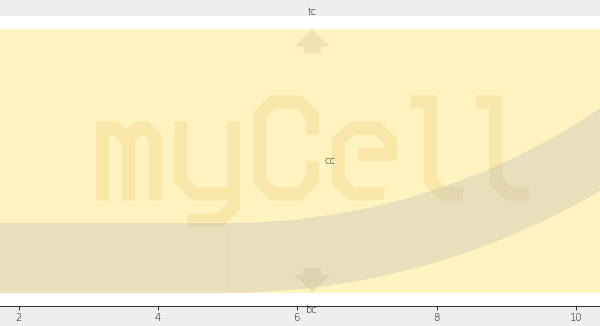In this example we show how to create xsections and layers